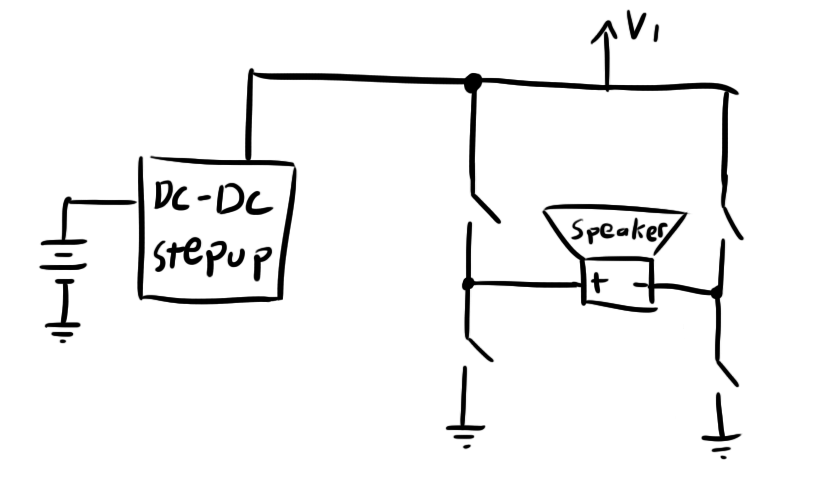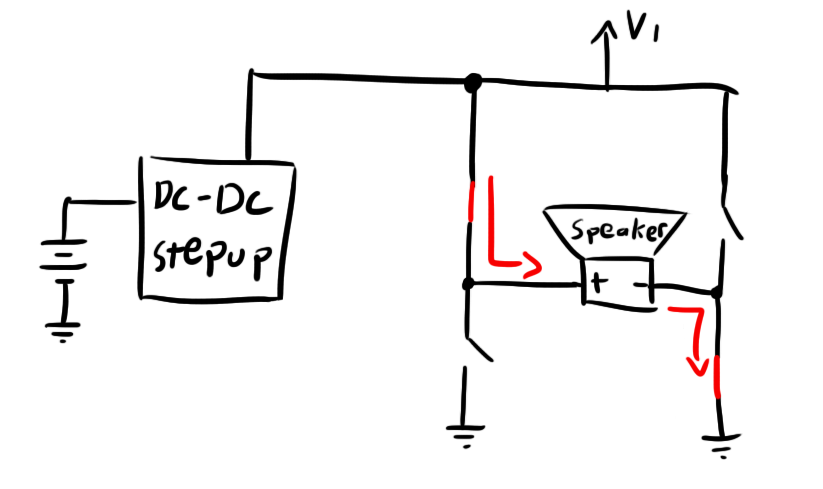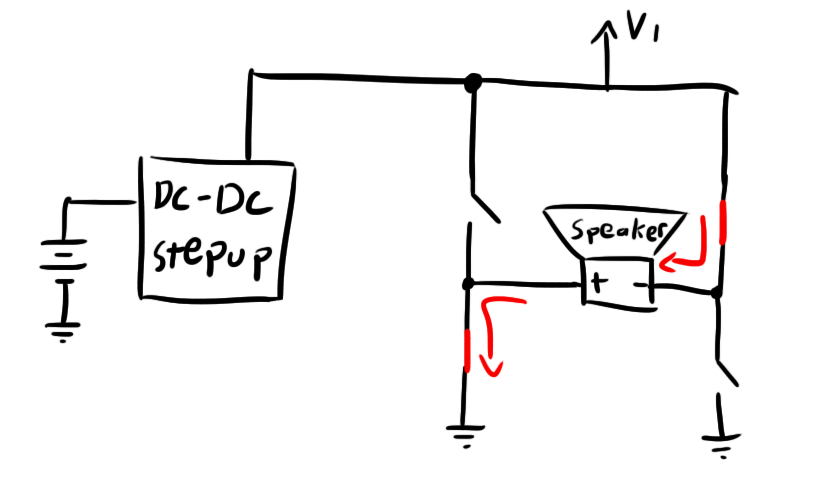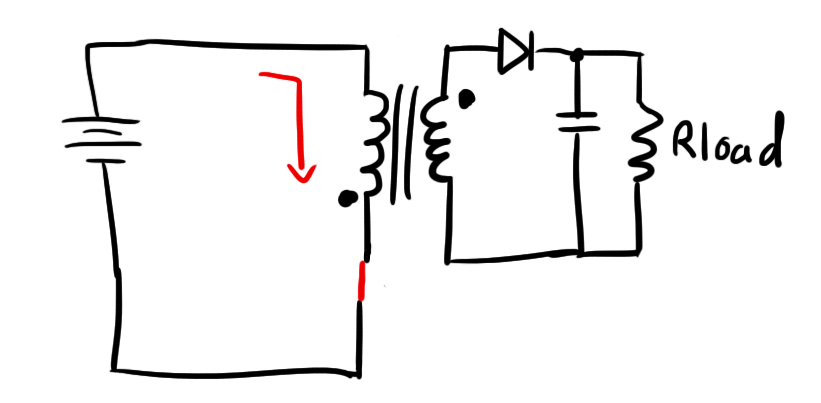# Theory

### Classy D

After discussing my predicament with some friends, it was suggested that I try doing it with a Class D amplifier.  Class Ds are often used in audio applications offering cheap and efficient audio amplification.  They can distort the audio a bit, so they’re not perfect, but I wasn’t really looking for a pristine output waveform with this project. Here’s an example of a Class D amplifier in an audio application:There are four switches driving the speaker in this case.  Depending on the configuration of these switches, you can drive current through the speaker either way:So, this is a good solution for me.  It’s especially enticing because it lets me drive the EL wire at a positive or negative voltage.

Switching transistors back and forth is a piece of cake.  I did it before for my wiper driver.  The only issue is how to get that +120V Vdd.

### If I Wanted My Fly Back…

A flyback converter is a kind of DC-DC voltage step-up converter that is commonly used in applications where you need to get a high output voltage from a low input voltage.  They are perhaps most commonly used in the flashes of cameras (that whining sound you’ve probably heard when charging up the flash on a crappy disposable camera).  They’re also used in situations where you need an DC supply that is entirely electrically isolated.  In my schematic, I ended up tying part of the output to ground, but there are several situations where you would want to remove all current paths between the main circuit and the output current loop, and a flyback lets you do that.

A flyback converter is kind of like a typical boost converter.  It stores energy in the magnetic field inside a transformer and then allows it to discharge at a different voltage/current.  Here’s a simple schematic.In this picture, the switch would probably be some kind of transistor as it needs to switch very quickly.  Let’s take a moment to figure out exactly how this thing works and how to determine the input/output voltage.

When the switch is closed, current starts flowing through the primary side of the transformer.  This current will ramp up linearly at a slope proportional to the inductance of the primary winding.Ideally, the secondary winding will induce a current that will fight the change of magnetic field (Lenz’s Law).  The dot notation on the transformer symbol helps to indicate current direction.  As current on the primary ramps up traveling “toward the dot”, current on the secondary will ramp up “away from the dot”.  As you can see, the diode will prevent this from happening.

As a result, the current in the primary winding ramps up and stores energy in the magnetic field inside that winding.

When the switch is opened, the result will be the current on the primary in the “toward the dot” direction ramping down (to zero).  The transformer will try to fight this by producing current “toward the dot” to compensate.  Because the primary is open, all of this induced current will happen in the secondary winding and toward the output.The diode passes this current toward the load, and the capacitor smooths that voltage.

### Now Some Numbers

So, let’s work out some math to figure out exactly what output voltage you can expect for a given input voltage, duty cycle, and winding ratio.  This should be fairly easy because there are two very distinct stages of operation and the only thing tying them together is the magnetic flux built up in the core.  Let’s start with the first stage where the magnetic field is ramping up.

The flux due to the primary winding will be:

$$\Large \Phi = \frac{\mu N_{1} i_{1} A}{l}$$

Where $$\mu$$ is the permeability of the core, $$N$$ is the number of windings, $$A$$ is the cross-sectional area of the core, and $$l$$ is the magnetic path length of the core.

Now, to get that flux, we need to let the current ramp up in the core.  We will start with the formula I introduced back here:

$$\Large V\times \Delta t = L \times \Delta i$$

Let’s say that our $$\Delta t$$ is equal to the amount of time per cycle that the switch remains closed.  This will be:

$$\Large \Delta t=\frac{D}{F}$$

The inverse of the frequency (F) gives you the total period of the cycle, and multiplying by the duty cycle (D) gives you the portion during which the switch is closed.

$$\Large V\times \frac{D}{F} = L \times \Delta i$$

So let’s solve for current (assuming initial current is zero or $$\Delta i = i$$).

$$\Large i = \frac{VD}{LF}$$

So putting it all together, we get:

$$\Large \Phi = \frac{\mu N_{1} AV_1 D}{lL_1 F}$$

Remember that:

$$\Large L=\frac{\mu N^2 A}{l}$$

So we can rewrite the above as:

$$\Large \Phi = \frac{\mu N_{1} AV_1 D}{lF}\times \frac{l}{\mu N_1^2 A}$$

$$\Large \Phi = \frac{V_1 D}{N_1 F}$$
So, we know that the flux is shared between the two windings.  Once the flux is built up by the primary, it’s allowed to discharge through the secondary.  We can rewrite the above for the secondary winding when the switch is opened:

$$\Large \Phi = \frac{V_2 (1-D)}{N_2 F}$$

Remembering that this is the state that takes up the rest of the time in the cycle ($$1-D$$).

Also note that because we’re considering a “steady state” condition here, all of the flux that builds up in the first part of the cycle must discharge during the second part.  Otherwise, you’d have some flux left over and you wouldn’t quite end up where you started.  Let’s set the fluxes equal to each other:

$$\Large \frac{V_2 (1-D)}{N_2 F}=\Phi = \frac{V_1 D}{N_1 F}$$

$$\Large \frac{V_2 (1-D)}{N_2}=\Phi = \frac{V_1 D}{N_1}$$

And solve for the output voltage:

$$\Large V_2 = \frac{D}{1-D} \frac{N_2}{N_1}V_1$$
Alright, that wasn’t too painful.  So, as you can see, you have a few options for how to get your output voltage.  Ideally, you could get an infinite output:input ratio with a 1:1 turns ratio, but that would require an insanely high duty cycle which is kind of hard to pull off with conventional components (usually don’t want to go anywhere past 90%) or so.

### Regulating a flyback

Regulating a flyback can be fairly difficult because the output voltage will depend on your load.  Keep in mind that we were making the assumption in the above calculations that all of the flux built up in the primary will dissipate in the secondary.  If the output load were to suddenly have a larger impedance, the secondary current would decrease, and it would take longer to dissipate the flux.  This means that all of the flux might not be dissipated by the end of the cycle.

When the next cycle comes around, this original flux will be added to the new flux and both will be discharged.  Because flux is proportional to current in the secondary winding at this point in the cycle, the output current will increase.  This increased current through the increased resistance will produce an increased output voltage.  To prevent this, the duty cycle must be reduced in an attempt to bring the current ripple back into the “barely touching zero” condition outlined in this section.

This is why it’s important to never operate a flyback converter with no load.  With infinite load resistance, the output voltage will continue to rise until it eventually breaks something.  This is actually how flyback converters in disposable cameras operate, but they depend on the leakiness of their cheap output capacitors to prevent the output voltage from getting too high.  Any additional charge will leak through the cap.

So whatever method is used to regulate the flyback converter must keep a close eye on the output.

So, before continuing, let’s talk about component sizing.

## 28 thoughts on “Dimming an EL panel is harder than it looks”

1.matthew katzenstein on said:

yea there is a MUCH MUCH easier way to do this..
you take the standard inverter and the el panel and put a CDS photocell in series then take a standatd lm328 amplifier and hook the output to a bicolor LED and shine that on the photocell
DONE! i can give you schematics and stuff if you want

•ch00f on said:

So you’re just talking about putting an adjustable resistance in series with the EL panel? I suppose that would work, but it’s not the most efficient way to do it.

Also, looking at a few datasheets, the maximum power dissipation though a CdS cell is around 100mW. If I were to use one on a panel, it might need to dissipate upwards of 7-800mW. It couldn’t take the heat. I suppose you could put a few in series, but that’s going to get pretty messy.

This solution might work in smaller loads like short EL wires though. Thanks for the tip!

•Graham Toal on said:

When I first read your comment above I thought you were using the photocell to read the light from the EL panel and then reverse the voltage polarity when it detected maximum brightness, which should generate AC that would self-regulate to the right frequency to sustain that brightness level. But then when you mentioned LEDs you lost me! But would a feedback control mechanism like what I thought you meant actually work?

•ch00f on said:

Matthew was recommending using a optoisolator as a variable resistance. The idea is that you could control current from a low-voltage circuit and not have to worry about the 120V blowing anything up.

You present an interesting idea, but I think it would end up being more trouble than it’s worth. The EL panel is a fairly “slow” material. It takes a few milliseconds for it to react to changes in current, so using its brightness in some kind of feedback system would prove difficult. To say nothing of the wiring/mechanical complications this would create.

2. >> I chose the 1N4148 which has a gate capacitance of just 4pF.

Not gate. Junction.

Since you were ordering from Digi-Key anyway, you could have purchased some BAT-41 Schottky diodes (19,000 in stock) . The BAT-41’s capacitance is 2pF, half of the 1N4148’s capacitance, and its forward voltage at 20mA forward current is 0.30 volts per diode, significantly less than the 1N4148’s 0.95 forward volts per diode.

•ch00f on said:

Whoops, typo. Thanks.

I figured out my diode problem after I already made my order, so I had to use what I had on hand. Next time I’ll be sure to choose more wisely. Thanks for the tip!

3.dcroy on said:

while pouring over various patents on zinc sulfide phosphors i discovered reference to the color shift in el wire, my 555 based driver was a quick and dirty means of observing it myself and as an excuse to learn something about driving el wire… more of a first attempt using spare parts before devoting more time to designing a proper circuit rather than basing it off some simple dc to ac inverter circuit i found in the internet

along with the brightness being a function of frequency i found voltage had a similar effect but would cause the el wire to age faster than driving at a higher frequency would, and yes driving at higher voltages also caused my salvaged transformers to overheat and leak paraffin wax everywhere

•ch00f on said:

Oh by all means, I think it’s great what you did, and it really helped me in my initial research! I was just making a point that in general, there aren’t too many resources available on EL wire and driving it properly.

•dcroy on said:

there really arent, i had to resort to reading dozens of white papers and patents to figure everything out, my result was a working driver that was extremely inefficient, occasionally overheated and administered ~130 volt shocks when i wasn’t paying attention

ive also been working out some ideas on mixed frequency driving el wire to increase lifespan while making it brighter by rapidly switching between low and high frequencies at a rate where it would be brighter while impacting lifespan al little as possible

4. I also noticed the frequency shift.
Wonder if this effect can be used to make a variable spectrum microscope light?
For certain applications this would be handy indeed as if the wavelength shift is linear then you could image a sample at a range of multispectral frequencies rather than green or blue.

I like the Supertex HV852 for this as it draws under 5mA with small wires allowing a PIC to directly drive it and adjust frequency with a single drive lead and a capacitor.
So one 12F683 can run six separate EL wires, with Charlieplexing even more.

•dcroy on said:

as far as i have observed there is only a single color shift at ~2000 hertz and i have not found any information to indicate there any more

it is far from linear and im not sure the amount of light would be sufficient for a microscope anyways

5.Marc Harris on said:

I found a great source for the EL panels. http://www.etsy.com/shop/lightkraft I looked all over and they are the least expensive in the US.. Most of their prices include the inverters (which are matched to each panel). Another good source is EL-WORKS http://www.el-w.com

6. Pingback: Bucking It Up » Geko Geek

7.Roberto Certain on said:

Hi,
How can I contact you? Email

•ch00f on said:

Just click the email button at the top of the page!